Convertible Bonds: Definition

A convertible bond is a type of bond that gives its holder the right to convert it into a fixed number of shares within the issuing company. A convertible bond is also known as a convertible note, convertible debt, or convertible debenture.

Convertible bonds are convertible into the firm’s common stock at some future specified date. For this reason, they enable the bondholder to obtain an equity interest in the firm eventually.

This conversion feature allows the firm to issue the bond at a lower interest rate.

Convertible bonds are usually callable. This means that the borrowers or issuer can call the bonds prior to their maturity. Thus, the bondholder is forced either to convert the bonds or to have them called prior to their maturity.

Convertible Bonds: Explanation

Convertible bonds have two potential effects on earnings per share (EPS).

First, if they are converted, the firm avoids the interest expense that it would have incurred.

Second, the number of common shares is increased by the conversion. The actual effect on reported EPS depends on whether they are considered to be common stock equivalents.

If they are equivalent, the accountant acts as if they were converted in calculating both primary and fully diluted EPS. If they are not considered equivalent, they are included only in the fully diluted EPS calculations.

Common Stock Equivalency of Convertible Bonds

In general, convertible bonds are equivalent to stock if the initial investors appear to have bought them for their convertibility, and if this intent is indicated by accepting a lower yield than they would have obtained had they simply bought bonds.

Specifically, a test calls for a comparison of the cash yield (cash interest payment divided by the issue price) of the bond with a cutoff rate. If the yield is less than the cutoff rate, the bonds are considered equivalent for as long as they are outstanding.

Example

Consider two groups of convertible bonds:

• Group A has a nominal rate of 6% and is sold for 98% of face value. Its cash yield (per \$100) is 6.12%(\$6 / \$98).
• Group B has a nominal rate of 11% and is sold for 107% of face value. Its cash yield (per \$100) is 10.28% (\$11 /  \$107). If the prime rate at the time of issuance is 12%, the cutoff rate is 8%.

Consequently, group A would be classified as equivalent to common stock but group B would not be considered to be equivalent.

Other tests that could have produced different results were rejected. These include:

• Yield tests using present value concepts
• Cutoff rates established for investments of comparable risk
• Comparison of the bond’s market value with the market value of the shares to which it could be converted.

It is worth noting that tests can be applied every year instead of only at the time of issuance. However, certain tests are too subjective and not sufficiently practicable.

Impact of Convertible Bonds on EPS

If the assumption is made that the bonds have been converted, an accountant should add to the denominator the number of common shares that would be issued if all the bonds were exchanged.

For the numerator, it is necessary to calculate the interest expense saved (including amortization) less the additional taxes that would have been paid.

For example, if \$92,000 in interest expenses would have been avoided and the tax rate is 45%, the numerator would increase by the after-tax amount of \$50,600 (i.e., \$92,000 less \$41,400).

For primary EPS calculations, conversion is assumed only for bonds that are both common stock equivalents and convertible within the next five years. For fully diluted EPS, conversion is assumed for all bonds that are convertible within the next 10 years.

Antidilution occurs when the net interest savings per potentially issuable common share exceeds the EPS computed without considering conversion.

For example, if EPS before conversion is \$12 per share, any net savings per new share in excess of \$12 will increase the average.

Example

Suppose that the Sample Company has three issues of convertible bonds outstanding. Each has a face value of \$10,000,000 and is convertible to 250,000 common shares.

The following facts are known about each group: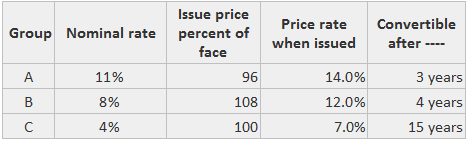The test for equivalency is shown below: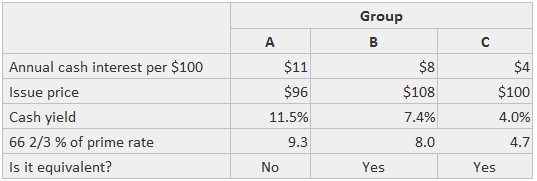The impact on the EPS denominators is presented in this table: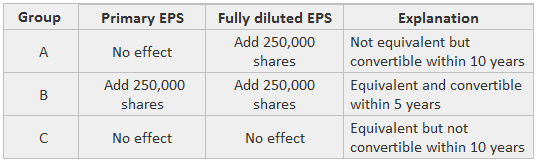The numerator is affected as follows (assuming straight-line amortization and 20-year lifetimes):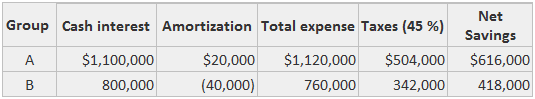The EPS calculations are shown below, including simple EPS. This shows the extent of dilution (the net income and outstanding shares are assumed):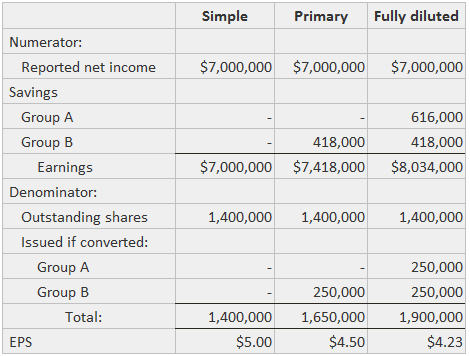Conversion or Issuance of Convertible Bonds During the Period

If convertible bonds are actually converted during the period, EPS figures are calculated as if the bonds were converted at the beginning of the period (this same assumption is made for bonds that are not converted).

However, the numerator is adjusted only for the interest costs (net of tax) that are actually incurred during the part of the year in which they were outstanding.

For newly issued convertible bonds, the numerator is adjusted only for the interest expense (net of tax) that is actually incurred during the part of the year in which they are outstanding.

The number of hypothetical common shares that would have been issued is weighted by the fraction of the year that the bonds were outstanding.

True is a Certified Educator in Personal Finance (CEPF®), contributes to his financial education site, Finance Strategists, and has spoken to various financial communities such as the CFA Institute, as well as university students like his Alma mater, Biola University, where he received a bachelor of science in business and data analytics.

To learn more about True, visit his personal website, view his author profile on Amazon, his interview on CBS, or check out his speaker profile on the CFA Institute website.